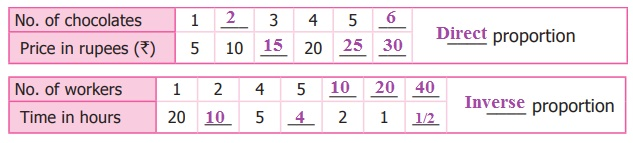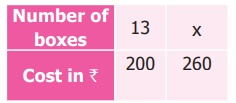Home | | Maths 7th Std | Inverse Proportion

# Inverse Proportion

The following table shows the number of workers and the number of days to finish the construction of water tank in school.

Inverse Proportion

Let us consider the following situation.

Situation

The following table shows the number of workers and the number of days to finish the construction of water tank in school.In this situation, the two quantities namely number of workers and the number of days are related to each other. We observe that when the number of workers increases, the number of days to finish the job decreases. Consider the number of workers as x and the number of days as y then we note that the product is always the same. That is,

x × y = 2 × 40 = 4 × 20 = 5 × 16 = 10 × 8

Consider each of the value of x and the corresponding value of y. Their products are all equal say xy = 80 = k (k is a constant) and it can be expressed as xy = k (k is a constant)

If y1 and y2 are the values of y corresponding to the values of x1 and x2 of x respectively then x1 y1 = x2 y2 (=k) x1/y1 = x2/y2 . We say that x and y are in inverse proportion.

Think

Think of an example in real life where two variables are inversely proportional.

Try these

1. Complete the table given below and find the type of proportion2. Read the following examples and group them in two categoriesActivity

Form all possible rectangles with area 36 sq.cm by completing the following table.(i) When length decreases, the breadth increases

(ii) When breadth increases, the length decreases

(iii) If the length is 8 cm what will be the breadth? -Discuss.

Extend this activity and try the same with area 24 and 48 sq.units.Example 4.5

60 workers can spin a bale of cotton in 7 days. In how many days will 42 workers spin it?

Solution

Let x be the required number of days. The decrease in number of workers lead to the increase in number of days. (Therefore, both are in inverse proportion)For inverse proportion x1y1 = x2y2

Hence 60 × 7 = 42 × x

42 × x = 60 × 7

x = 60×7 / 42

x = 10

In 10 days 42 workers can spin a bale of cotton.

Example 4.6

The cost of 1 box of tomato is 200. Vendan had money to buy 13 boxes. If the cost of the box is increased to 260 then how many boxes will he buy with the same amount?

Solution

The cost of one box = ₹ 200

Increased cost of one box = ₹ 260

Let x be the number of boxes bought by Vendan.

As cost of boxes increases the number of boxes decreases.This is in inverse proportion. Therefore, x1y1 = x2y213 × 200 = x × 260

x × 260 = 13 × 200

x = [13 × 200] /  260

x = 10

Therefore, he can buy 10 boxes for the same amount.

Direct and indirect proportion is very much useful in project scheduling. A project can be any work that is time bound like building a house, construction of bridges, etc.Tags : Term 1 Chapter 4 | 7th Maths , 7th Maths : Term 1 Unit 4 : Direct and Inverse Proportion
Study Material, Lecturing Notes, Assignment, Reference, Wiki description explanation, brief detail
7th Maths : Term 1 Unit 4 : Direct and Inverse Proportion : Inverse Proportion | Term 1 Chapter 4 | 7th Maths